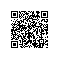# java学习笔记10--泛型总结

## 集合类中的数据类型

Vector v=new Vector();
String name=(String)v.get(0);  //强制类型转换，OK

// 由于Date类型不能转换为String，下面语句会在运行时发生错误，但这种错误在编译时不会被检查出来
String date=(String)v.get(1); //编译器不会发现这里有问题

• 强类型集合类中，只能存储指定类型的数据

• 在强类型集合类中取出数据时，无需进行类型转换处理，如果数据类型不配备，编译时会直接报错

• 强类型集合并没有引入新的类名，只需在定义原有集合对象时，用尖括号(<>)指明其存储的数据类型名称即可。

//下面的向量类的实例中只能存储字符串类型数据
Vector<String> v=new Vector<String>();
String name=v.get(0); //取出时，无需做类型转换,如果想在这种强类型集合中加入日期数据，在编译时就会报告错误
v.add(new Date()); //编译器会直接报告类型不匹配错误

## 泛型类

 [访问修饰符]  class 类名<泛型1,泛型2,…> {
泛型1  泛型成员1;
泛型2  泛型成员2;
//....
}

//此处声明了一个包含泛型T的泛型类,T代表所有可能的类型，而T的实际类型在Generic类实例化时指定。
class Generic<T> {
private T f;  //f为泛型成员
public void setF(T f) {//setF方法的参数类型为泛型T
this.f = f;
}
public T getF() {//getF方法的返回类型为泛型T
return f;
}
}

public class test {
public static void main(String args[ ]) {
//f1中的泛型T在此指定为Boolean类型
Generic<Boolean> f1 = new Generic<Boolean>();

//f2中的泛型T在此指定为Integer类型
Generic<Integer> f2 = new Generic<Integer>();

//f1的setF方法只能接受Boolean类型的数据
f1.setF(new Boolean(true));
Boolean b = f1.getF();
System.out.println(b);

//f2的setF方法只能接受Integer类型的数据
f2.setF(new Integer(10));
Integer i = f2.getF();
System.out.println(i);
}
}

Generic f3 = new Generic();
f3.setF(new Boolean(false));

Note: Generic.java uses unchecked or unsafe operations.

Note: Recompile with -Xlint:unchecked for details.

Generic<String>[] gs;  //声明泛型类的数组

//先对泛型数组进行初始化
gs = new Generic; //不要写成new Generic<String>

//再分别为每一个数组元素进行初始化
gs = new Generic<String>();//为第一个数组元素赋值
//....

class Generic2<T1, T2> {
private T1 f1;
private T2 f2;
//...
}

//给出泛型T1， T2的实际类型
Generic<Integer, Boolean> f = new Generic<Integer, Boolean>();

//没有给出泛型T1， T2的实际类型
Generic f1 = new Generic(); //T1， T2将默认为是Object类型

class Generic<T> {
private T[] array;    //此处不能用new T[]实例化array
public void setArray(T[] array) {
this.array = array;
}
public T[] getArray() {
return array;
}
}

public class test {
public static void main(String args[ ]) {
String[] strs = {"red", "black", "green"};
Generic<String> f = new Generic<String>();
//向泛型成员array传递实际的字符串数组
f.setArray(strs);
//读取泛型成员array的值，将其赋给字符串数组变量strs
strs = f.getArray();
}
}

class Generic<T>{
T f;
void setF(T f){ this.f = f; }
//....
void doSome(){
//getClass和toString都是Object中的方法
System.out.println(f.getClass().getName());
System.out.println(f.toString());
}
}

public class javatest {
public static void main(String args[ ]) {
String strs = "hello";
Generic<String> f = new Generic<String>();
f.setF(strs);
f.doSome();
}
}

## 限制泛型上限类型

extends关键字用来指定泛型的上限，在实例化泛型类时，为该泛型指定的实际类型必须是指定类的子类或指定接口的子接口

import java.util.List;
public class ListGeneric<T extends List> {
private T list;
public void setList(T list) {
this.list = list;
}
public T getList() {
return list;
}
}

ListGeneric<Vector> f1 = new ListGeneric<Vector>();
ListGeneric<ArrayList> f2 = new ListGeneric<ArrayList>();

ListGeneric<HashMap> f3 =  new ListGeneric<HashMap>();
type parameter java.util.HashMap is not within its bound
ListGeneric<HashMap> f3 = new ListGeneric<HashMap>();

class Generic<T> {
//...
}

class Generic<T> extends Object {
//...
}

class ListGeneric<T extends List>{
private T list;
public void setList(T list) {
this.list = list;
}
public void doSome() {
System.out.println(list.get(0));
}
}

Object是所有类的父类，因此，所有的类型的实例都可赋值给声明为Object类型的变量

Boolean f1 = new Boolean(true);
Integer f2 = new Integer(1);
Object f = f1;    //ok
f = f2;        //ok

Generic<Boolean> f1 = new Generic<Boolean>();
Generic<Integer> f2 = new Generic<Integer>();
Generic<Object> f=f1; //f1和f类型并不兼容,发生编译错误
f=f2;  //f2和f类型同样不兼容，也会发生编译错误 

## 泛型通配字符（Wildcard）

Generic<Boolean> f1 = new Generic<Boolean>();
Generic<Integer> f2 = new Generic<Integer>();
Generic<Object> f3 = new Generic<Object>();
Generic<?> f;
f = f1;    //ok
f = f2;    //ok
f = f3;    //ok

class test {
//Collection<?>可以匹配任意强类型的集合
static void printCollection(Collection<?> c) {
for(Object o : c)
System.out.println(o);
}
}

Generic<? extends List> f = null;
f = new Generic<ArrayList>();    //ok
//...
f = new Generic<Vector>();    //ok
//...
//以下语句会发生编译错误，因为HashMap没有实现List接口
f = new Generic<HashMap>();
incompatible types
found : Generic<java.util.HashMap>
required: Generic<? extends java.util.List>
f = new Generic<HashMap>();

//将f限定为只能代表采用java.sql.Date的父类实例化的
Generic<? super java.sql.Date> f = null;
f = new Generic<java.sql.Date>();    //ok

//OK，java.util.Date是java.sql.Date的父类
f = new Generic<java.util.Date>();

//错误，因为String不是java.sql.Date的父类
f = new Generic<String>();

## 泛型方法

实现代码

}

class cc{
/*
方法fun声明了一个泛型T，该方法将任意类型的数组a中的所有
元素复制到相应的强类型集合c当中而不会导致编译错误。
此处的泛型声明T仅作用于fun方法的声明部分和实现代码部分。
*/
public static <T> void fun(T[] a, Collection<T> c){
for(T o : a)
}
}

//对cc中定义的泛型方法fun进行调用测试
public class javatest {
public static void main(String args[ ]) {
String[] sa = new String;
Collection<String> cs = new Vector<String>();
Collection<Object> co = new Vector<Object>();

cc.fun(sa, cs);    //fun中的泛型T此时匹配类型String
cc.fun(sa, co);    //fun中的泛型T此时匹配类型Object
}
}

class cc{
//限定aToC方法中的泛型T必须是实现了序列化接口的类型
public static <T extends java.io.Serializable> void fun(T[] a,Collection<T> c){
for(T o : a)
}
}

## 原始类型和向后兼容

public class GenericStack<E> {
ArrayList<E> list = new ArrayList<E>();
public int getSize() {
return list.size();
}
public E peek() {
return list.get(getSize() - 1);
}
public void push(E o) {
}
public E pop() {
E o = list.get(getSize() - 1);
list.remove(getSize() - 1);
return o;
}
public boolean isEmpty() {
return list.isEmpty();
}
}

GenericStack stack = new GenericStack();

//大体等价于于下面的语句
GenericStack<Object> stack = new GenericStack<Object>();

class Max {
public static Comparable max(Comparable o1, Comparable o2) {
if(o1.compareTo(o2) > 0)
return o1;
return o2;
}
}

public class javatest {
public static void main(String args[]) {
Max.max("welcome", 12);
}
}

Exception in thread "main" java.lang.ClassCastException: java.lang.Integer cannot be cast to java.lang.String

class Max {
public static <E extends Comparable<E>> E max(E o1, E o2) {
if(o1.compareTo(o2) > 0)
return o1;
return o2;
}
}

## 继承中的泛型

class subGeneric<T1, T2, T3> extends Generic<T1, T2> {
private T3 f3;
public void setF3(T3 f3) {
this.f3 = f3;
}
public T3 getF3() {
return f3;
}
}

public class SubGeneric<T3> extends Generic<String, Object> {
private T3 f3;
public void setF3(T3 f3) {
this.f3 = f3;
}
public T3 getF3() {
return f3;
}
}

## 泛型接口

interface I<T1, T2> {
T1 getT1();
T2 getT2();
//...
}

class IC implements I {
public Object getT1() { }
public Object getT2() { }
//...
}

class IC<T1, T2> implements I<T1, T2> {
public T1 getT1() { }
public T2 getT2() { }
//...
}

I<String, Integer> i = new IC<String, Integer>();

interface I<T1, T2> {
T1 getT1();
T2 getT2();
//...
}
//实现接口I时，直接指定泛型T1、T2的类型
class IC implements I<String, Integer> {
//由于指定接口I中T1类型为String，getT1返回类型必须为String
public String getT1() { }
//由于指定接口I中T2类型为Integer，getT2返回类型必须为Integer
public Integer getT2() { }
//...
}

## 泛型和枚举

public enum TrafficLight{
Red,Amber,Green;
private int duration;
public static <T> void avgDuration(Collection<T> carType){
//....
}
//....
}使用钉钉扫一扫加入圈子
+ 订阅